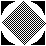Inductive Solutions, Inc.
380 Rector Place, Suite 4A, New York, New York 10280

Email  Telephone: +1 (212)945.0630   Fax: +1 (212)945.0367

Home

Products and Services
Software Products
Recommended Books

Bibliography and White Papers

## NNetSheet (This implementation was retired in 2017)

Neural Network FAQ
NNetSheet, a C-implementation of many popular neural network learning algorithms, helps build applications for data clustering, pattern recognition, and expert system rule generation. Algorithms for supervised learning (perceptron, delta rule, generalized delta rule with back propagation) and unsupervised learning (Hamming, Fuzzy Hamming, and Euclidean clustering) are provided.

Using Microsoft Excel as the user interface, NNetSheet implements neural network algorithms as fast dynamic link libraries for IBM compatible computers, and as code resources for Macintosh computers. All NNetSheet commands are configured in an easy-to-use Excel menubar that is integrated with all standard Excel commands and charting utilities. NNetSheet provides interactive help that takes you through the process of building neural network spreadsheet applications.

### System Requirements

NNetSheet requires Microsoft Excel.

### Input/Output

NNetSheet inputs are any set of training examples that can be read by Microsoft Excel in any one of 13 formats. NNetSheet "outputs" are neural network formulas. The formulas access the NNetSheet Dynamic Link Libraries. To use the neural network, simply cut and paste the formulas to an application.

### Size and Speed

The Excel spreadsheet limits the size of the training database to 32,787 rows and 256 columns. Training speed and neural network run-time speed depend on the hardware support for floating point computation.

NNetSheet supports the following algorithms and utilities:

• Perceptron
Learns functions that map input vectors to discrete target values.
• Delta Rule
Learns functions that map vectors to real target values.
• Generalized Delta Rule
Learns functions that map intervals to interval targets .
• Euclidean Clustering
Discovers data groupings based on Euclidean distances.
• Hamming Clustering
Discovers data groupings of bit-vectors.
• Fuzzy Hamming Clustering
Discovers data groupings of interval vectors.
• Hamming Histogram
Discovers data correlations of bit-vectors.
• Randomization
Improves convergence and separability by using nonlinear random inputs as a hidden layer.
• Autoscaling
Improves convergence by normalizing inputs and outputs.
• Representation of Text
Allows the NNetSheet-C algorithms to process text values, by mapping text to bit vectors.
• Log Returns
A collection of time series analysis tools used for random walk statistics.
• Moving Averages
A time series analysis tool used for smoothing and prediction.

The basic single-user license includes the neural network algorithm development tools integrated with the Microsoft Excel user inteface macros and dynamic link libraries; an on-line tutorial; a 120 Page Manual; and sample Applications. It comes with EMail Technical Support.

## Neural Network and NNetSheet FAQ

### What are Neural Networks?

Neural networks are special computing elements that can be trained to make decisions based on historical data. They work by discovering patterns and by inducing rules that define empirical relationships. Some example applications of neural networks include credit screening, stock market forecasting, bond rating, and market segmentation.

### Are Neural Networks Different From Expert Systems?

In rule-based expert systems, a knowledge engineer must deduce expert rules. Neural networks induce rules directly from historical data in the form of pattern recognition functions. You can use these functions just like the classification rules in an expert system.

### How do Neural Networks Compare with Statistical Techniques?

Neural network supervised learning corresponds to statistical non-linear discriminant analysis; neural network unsupervised learning corresponds to statistical clustering and factor analysis. Instead of executing complex statistical procedures -- the neural network learning model iteratively converges to a pattern recognition function that is a optimal in a least-mean-square sense. The iterative process implicitly estimates the statistics of the historical training data. This parallels human pattern recognition: people learn patterns over time.

### What is NNetSheet?

NNetSheet is a development system that creates spreadsheet models of neural networks. We call these models Neural Network Spreadsheets. NNetSheet-C implements the neural network learning algorithms as dynamic link libraries -- C programs dynamically linked to Microsoft Excel spreadsheets. To the spreadsheet user, these neural network algorithms look like ordinary spreadsheet formulas.

### How is NNetSheet Different from other Neural Network Tools?

Our neural network tools maximize ease of use, comprehensiveness, and portability. The neural network models and preprocessing utilities include:

• Supervised Learning (for Prediction)
Perceptron Learning, Delta Rule, Generalized Delta Rule, Back Propagation
• Unsupervised Learning (for Clustering)
Hamming Metrics, Fuzzy Metrics, Hamming Histograms, Euclidean Metrics
• Data Preprocessing
Randomizing, Text Input, Scaling, Moving Averages

### How Easy is it to Build a Neural Network Spreadsheet with NNetSheet?

If you know how to use a spreadsheet, then you know how to use the NNetSheet. Building a neural network spreadsheet is a three step process:

1. Get the data for your problem and specify the learning.
Import data containing your training cases. Specify the learning or clustering required by selecting regions on the spreadsheet with the mouse.
2. Train the Neural Network Spreadsheet.
Set the number of epochs for training, the change tolerance, and other parameters. Run the neural network with the Calculate Now... spreadsheet command. The learning process is done when the weights converge.
3. Deploy and Customize.
Deploy the Neural Network Spreadsheet -- a spreadsheet that embeds the neural network as a set of spreadsheet formulas. Customize it -- just like any other spreadsheet -- and use the neural network formulas with the NNetSheet runtime system in your application.